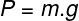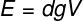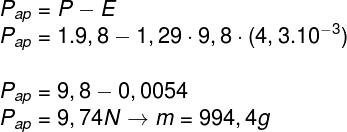Physics trivia

# Which is heavier: 1 kg of lead or 1 kg of cotton?

Both 1 kg of lead and 1 kg of cotton have the same mass and, consequently, the same weight. However, the measurement on the scale shows otherwise.

What are mass and weight?

Mass is a physical quantity inherent in bodies – it measures the amount of matter contained in them. The unit of mass in the International System of Units is the kilogram (kg).

Weight is the gravitational force of attraction that two masses exert on each other. Bodies with large masses, such as planets and stars, have a large gravitational field and therefore attract other bodies around them with greater force. Two identical bodies on different planets will always have the same mass, but their weight may differ, depending on the value of local gravity .

The weight of a body of mass m , subjected to a gravitational acceleration g , can be calculated by the following equation:Therefore, an object of mass 1 kg in the Earth’s gravitational field, which is approximately 9.8 m/s² , has a weight of 9.8 N:## Who is the heaviest?

After all, if the weights of 1 kg of lead and cotton are the same, why can scales indicate that cotton is lighter?

This happens because the scales do not directly measure the weight of bodies , but the reaction to compression , called the normal force .

If, when stepping on the scale, someone leans on you, making a downward force , it will indicate a greater mass . However, the increase indicated by the balance is not related to an increase in mass , but to the increase in the compression force applied to it.

Similarly, when we place 1 kg of cotton on a scale, it measures a smaller compressive force than that exerted by 1 kg of lead . This is because all bodies that are inside a fluid such as water or atmospheric air are subject to a vertical force, which points upwards, called buoyancy .

The buoyancy arises on bodies that occupy space within a fluid as a result of the pressure difference (atmospheric or hydrostatic). Pressure, in turn, is closely related to height: the greater the depth , the greater the influence of the pressure exerted on the body – therefore, the lower portions of a body suffer greater pressure . If the resultant of the forces acting on the body points upward, it will float, just as bubbles of gas in a soda or a hot air balloon do.

Pressure on the lower parts of the body is greater than the pressure above, causing it to float

The buoyancy is a vector quantity that depends on the density of the fluid, the local gravity and the volume of displaced fluid, and can be calculated by the equation below:The resultant of the weight and buoyancy forces is called the apparent weight . It is thanks to this net force that things appear lighter than they really are when they are in the water, for example. The greater the volume of the body , the smaller its apparent weight , which is precisely why cotton will have a slightly smaller measurement on the scale than lead. To have the same mass as lead , cotton takes up a much larger volume . , since its density is low . As a result, the thrust on it is much more significant than that exerted on lead .

## Calculating the buoyancy on cotton

Let’s calculate the apparent weight of 1 kg of cotton, considering that: this cotton mass occupies a volume of 4.3 liters ( 4.3 x 10 -3 m³), ​​the air density is approximately 1.29 kg/m³ and the local gravity is 9.8 m/s²:As the apparent weight of 1 kg of cotton is 9.74 N, the balance would indicate a mass of 994.4 g of cotton.

Since it is very dense , lead occupies a much smaller volume – so the atmospheric buoyancy on it is almost negligible and hardly measured by conventional balances, which have low accuracy.